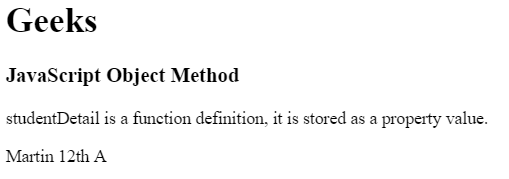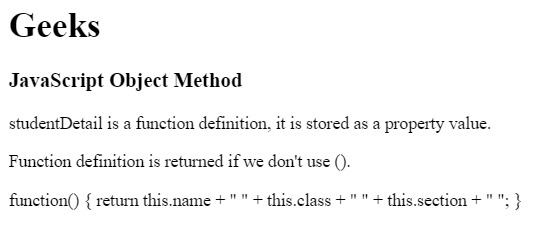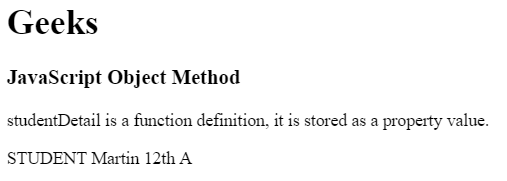Related Articles

# JavaScript | Object Methods

• Last Updated : 04 Aug, 2021

Object Methods in JavaScript can be accessed by using functions. Functions in JavaScript are stored as property values. The objects can also be called without using bracket ().

• In a method, ‘this’ refers to the owner object.
• Additional information can also be added along with the object method.

Syntax:

`objectName.methodName()`

Properties: A function may be divided into different property values, which are then combined and returned together.
For Ex: Student function contains the properties:

• name
• class
• section

Return Value: It returns methods/functions stored as object properties.
Example 1: This example use function definition as property value.

## HTML

 ```<``html``>` `<``head``>``    ``<``title``>``        ``JavaScript Object Methods``    ````` `<``body``>``    ``<``h1``>Geeks``    ` `    ``<``h3``>JavaScript Object Method``    ` `    ` `<``p``>``        ``studentDetail is a function definition,``        ``it is stored as a property value.``    ``` `    ` `    ``<``p` `id``=``"gfg"``>` `    ` `    ``<``script``>``    ` `        ``// Object creation``        ``var student = {``            ``name: "Martin",``            ``class : "12th",``            ``section : "A",``            ` `            ``studentDetails : function() {``                ``return this.name + " " + this.class``                    ``+ " " + this.section + " ";``            ``}``        ``};``        ` `        ``// Display object data``        ``document.getElementById("gfg").innerHTML``                ``= student.studentDetails();``    ````` `                               `

Output:Example 2: This example use storing property values and accessing without bracket ().

## HTML

 ```<``html``>` `<``head``>``    ``<``title``>``        ``JavaScript Object Methods``    ````` `<``body``>``    ``<``h1``>Geeks``    ` `    ``<``h3``>JavaScript Object Method``    ` `    ` `<``p``>``        ``studentDetail is a function definition,``        ``it is stored as a property value.``    ``` `    ` `    ` `<``p``>``        ``Function definition is returned``        ``if we don't use ().``    ``` `    ``<``p` `id``=``"gfg"``>` `    ` `    ``<``script``>``    ` `        ``// Object creation``        ``var student = {``            ``name: "Martin",``            ``class : "12th",``            ``section : "A",``            ` `            ``studentDetails : function() {``                ``return this.name + " " + this.class``                    ``+ " " + this.section + " ";``            ``}``        ``};``        ` `        ``// Display object data``        ``document.getElementById("gfg").innerHTML``                ``= student.studentDetails;``    ````` `                               `

Output:Example 3: Using function definition as property value and accessing with additional details.

## HTML

 ```<``html``>` `<``head``>``    ``<``title``>``        ``JavaScript Object Methods``    ````` `<``body``>``    ``<``h1``>Geeks``    ` `    ``<``h3``>JavaScript Object Method``    ` `    ` `<``p``>``        ``studentDetail is a function definition,``        ``it is stored as a property value.``    ``` `    ` `    ``<``p` `id``=``"gfg"``>` `    ` `    ``<``script``>``    ` `        ``// Object creation``        ``var student = {``        ``name: "Martin",``        ``class : "12th",``        ``section : "A",``        ` `        ``studentDetails : function() {``            ``return this.name + " " + this.class``                ``+ " " + this.section + " ";``        ``}``    ``};``    ` `    ``// Display object data``    ``document.getElementById("gfg").innerHTML``        ``= "STUDENT " + student.studentDetails();``    ````` `                                   `

Output:Supported Browser: